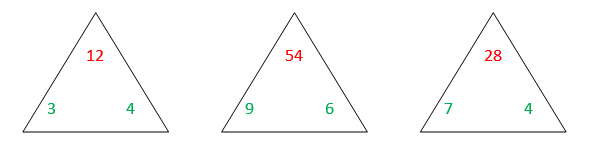# A Different Angle on Basic Facts

Many kids who struggle to learn their basic facts often believe there are hundreds of facts to be learned because they do not understand the relationship between the facts and between the operations.  A tool that can help students learn their facts while at the same time strengthening their understanding of the operations is triangular flash cards.

Give children a stack of old manila folders or index cards and have them cut out triangles that measure roughly 2 or 3 inches on each side. Round the corners to keep them from becoming dog-eared.

Multiplication/Division Examples:In the corners of the cards, students write the factors on the bottom two corners and the product on the top.  Students should use a different color for the products than they use for the factors.Students write the sum at the top of the triangle and write the two addends in a different color on the bottom two corners.

You may also use this template instead of cutting out triangles.

Once students create their own triangular flash cards, they can practice solving for the missing number in the fact family.  For example, the top left card (multiplication) above would represent the number sentences:      3 x 4 = 12, 4 x 3 = 12, 12 ÷ 4 = 3, 12 ÷ 3 = 4

The students can work in pairs or alone.  One child can cover any number on the triangle and the other child, thinking about the two other numbers, must state the covered number.  Students can then state the four facts (2 multiplication and 2 division) that are true for the 3 numbers in the triangle fact family

This reinforces not only multiplication facts, but also the fact that multiplication and division are inverse operations.  This helps students become familiar with vocabulary such as factors, sums, differences, products, etc.  When students construct their own triangular flash cards, they reinforce their understanding and learning.  They also have ownership of their cards.

Check out this video to learn more about how to use the cards: